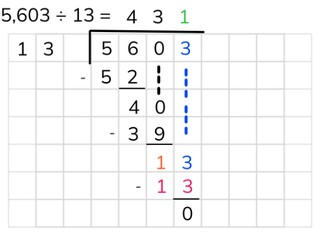Long division with a number greater than 1,000

# Long division with a number greater than 1,000

Students learn long division with a number greater than 1,000.8,000 schools use Gynzy92,000 teachers use Gynzy1,600,000 students use Gynzy

## General

The students learn long division with a number greater than 1,000.

## Standards

CCSS.Math.Content.6.NS.B.2

## Learning objective

Students will be able to use long division to divide a number greater than 1,000, without remainder.

## Introduction

The students solve the three division problems. Ask what steps they used to do this.

## Instruction

Explain that in long division you put the numbers of the problem next to one another. First you write the divisor on the left. Then you write the dividend on the right and draw a box around it. You will put the answer on top of the box. Show that you first look at the first digit of the dividend. That is the 5 of 5603. You cannot divide this 5 by 13, so you also need the second digit. Next you see how much you can divide 56 by 13. Emphasize that you can make a row of the table of 13 to help you with this. In this way you can quickly see what the largest number is that you can take away. In this case the largest number is: 4 × 13 = 52. Write the number 52 under the 56 and subtract. You write the answer (4) underneath. You write the 4, from 4 × 13, in the answer on top of the box. 13 does not fit into 4, so you look again at the next digit. Write the 0 from 5603 next to the 4. Now you have 40. Now you look again to see what the largest number is that you can take away. That is 3 × 13 = 39. Write the 39 under the number 40 and subtract them from each other. You now have 1 left over. You cannot fit 13 into 1. So you write the 3 from 5603 next to the 1. Now you have 13. The largest number that you can take away now is 1 × 13. Subtract the 13 from the 13. There is now 0 left over. Write the 1 next to the 3 in the answer. Your full answer is now 431. Next you can practice the problem 8656 ÷ 4 = together with the students. You can remove the cover to show the helping row. Now have the students solve the following problem on their own. Next you solve the problem with a number greater than 10,000 together with the students. Then you have the students solve the problem 15,225 ÷ 725 on their own.

Check whether the students can use long division to divide a number greater than 1,000 by asking the following question:
- What steps do you follow to solve the problem 1275 ÷ 25 using long division?

## Quiz

The students test their understanding of long division with a number greater than 1,000 through ten exercises. In these exercises, they must solve the division problems without remainder using long division. Two of the exercises are division problems with a number greater than 10,000.

## Closing

Discuss once again the importance of being able to use long division to divide a number greater than 1,000. As a closing activity you can have the students solve the problems in pairs. Have them first solve the problem independently and then check it with their partner. Also have them determine whether the problem could have been solved using fewer steps. After this you have the students come up with a division problem without remainder together with their partner. Then they trade problems to solve with another pair of students.

## Teaching tips

Have students that have difficulty with long division with a number greater than 1,000 first practice long division with a number to 1,000. Have these students first make a helping row, before they begin their calculations.

## Instruction materials

Scrap paper

### The online teaching platform for interactive whiteboards and displays in schools

• Save time building lessons

• Manage the classroom more efficiently

• Increase student engagement